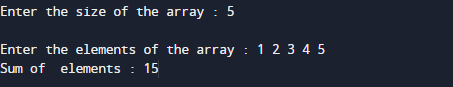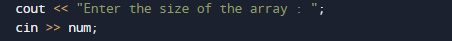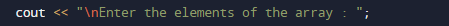# C++ Programs to Sum of Array Elements

In this tutorial you will learn about the C++ Programs to Sum of Array Elements and its application with practical example.

In this tutorial, we will learn to create a C++ program that will Calculate Sum of array elements using C++ programming.

## Prerequisites

Before starting with this tutorial we assume that you are best aware of the following C++ programming topics:

• Operators.
• looping statements.
• Basic input/output.
• Basic c++ programming
• For Loop.
• String.
• Array.

## What is array?

An Array is a collection variable elements  that can store multiple values in a single variable of same type.In Computer Science,an array is a data structure, or simply an array, is a data structure consisting of a collection of elements.

## C++ Programs to Sum of Array Elements

In this program we will add array elements. We would first declared and initialized the required variables. Next, we would prompt user to input elements of array .Later we add all the elements of Array.

## OutputIn the above program, we have first declared and initialized a set variables required in the program.

• a[] = it will hold entered elements.
• sum =hold sum or elements.
• i = for iteration.
• num= number of elements in array.

In our program, we will take value form user in array , after taking values we will add elements of an array.
First of all we  take size of an array from user in variable numafter taking size of array user will prompted to enter the elements of array.The variables ‘sum’ is initialized as 0.Then asked user to enter the array elements.Within the loop, the elements are stored in the array ‘=a[]’.
And in next loop the sum is calculated and stored in sum.As shown in below image.in every iteration. sum is calculated ans stored in variable sum  .At the end the value of sum will be printed.In this tutorial we have learn about the C++ Programs to Sum of Array Elements and its application with practical example. I hope you will like this tutorial.